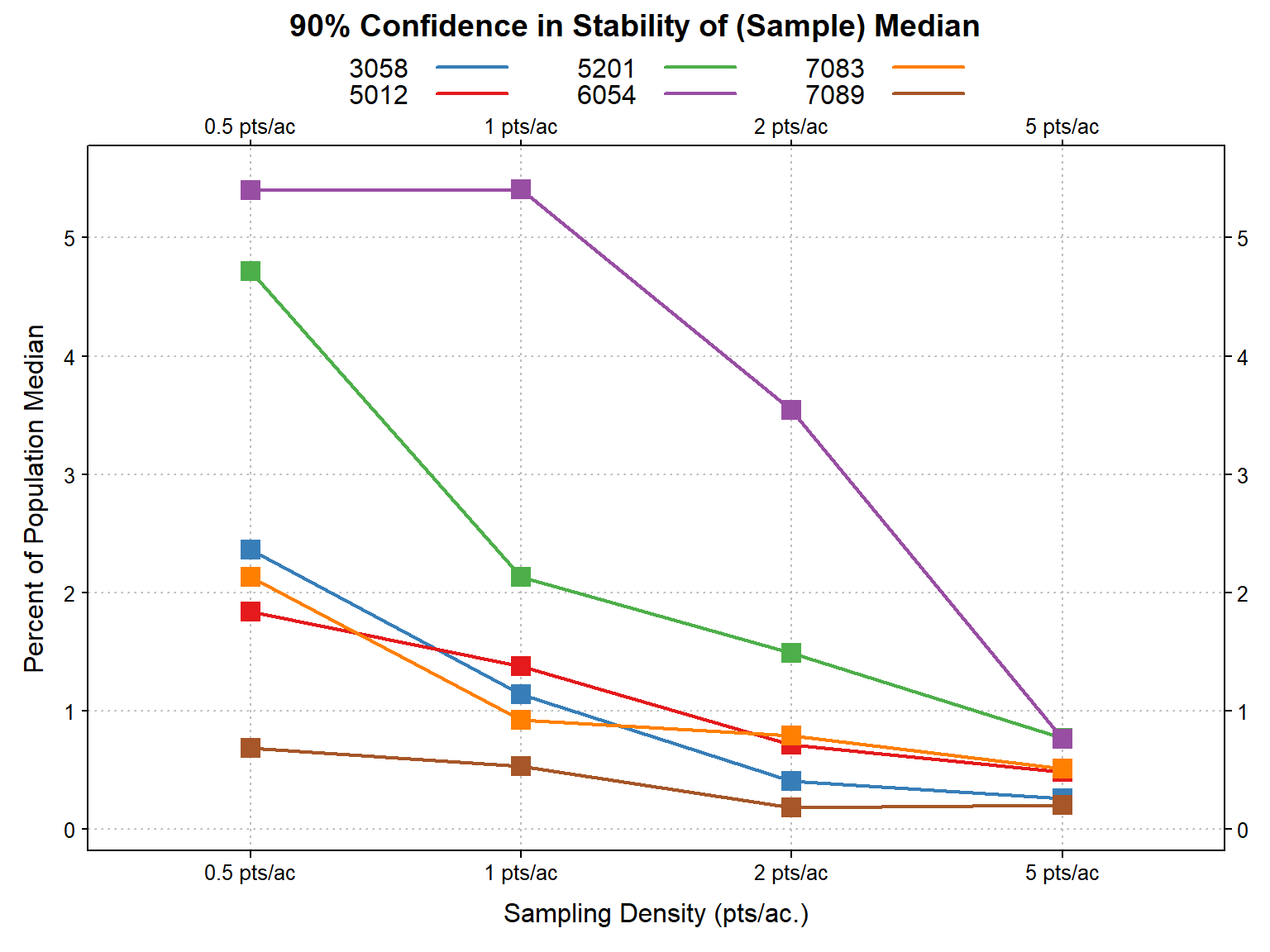The following can be used to evaluate the influence of sampling density on the stability of estimates derived from a raster data source. Adjust `mu` (map unit polygons), `mu.set` (select map unit symbols), and `r` (raster data source) accordingly.

``````library(latticeExtra)
library(tactile)
library(plyr)
library(rgdal)
library(raster)
library(sharpshootR)
library(parallel)

mu <-  readOGR(dsn='E:/gis_data/ca630/FG_CA630_OFFICIAL.gdb', layer='ca630_a', stringsAsFactors = FALSE)``````
``````## OGR data source with driver: OpenFileGDB
## Source: "E:\gis_data\ca630\FG_CA630_OFFICIAL.gdb", layer: "ca630_a"
## with 6623 features
## It has 11 fields``````
``````# best possible scenario: rasters are in memory

# subset to just those map units we are interested in
mu.set <- c('5012', '5201', '7089', '3058', '6054', '7083')
mu <- mu[mu\$MUSYM %in% mu.set, ]

# add a unique polygon ID
mu\$pID <- seq(from=1, to=length(mu))

# split select map units into a list of SPDF objs
# will be processed in parallel
mu.list <- split(mu, mu\$MUSYM)

# init cluster
cl <- makeCluster(4)
setDefaultCluster(cl)

clusterEvalQ(NULL, {
library(raster)
library(sharpshootR)
})``````
``````## []
##   "sharpshootR" "raster"      "sp"          "stats"       "graphics"    "grDevices"
##   "utils"       "datasets"    "methods"     "base"
##
## []
##   "sharpshootR" "raster"      "sp"          "stats"       "graphics"    "grDevices"
##   "utils"       "datasets"    "methods"     "base"
##
## []
##   "sharpshootR" "raster"      "sp"          "stats"       "graphics"    "grDevices"
##   "utils"       "datasets"    "methods"     "base"
##
## []
##   "sharpshootR" "raster"      "sp"          "stats"       "graphics"    "grDevices"
##   "utils"       "datasets"    "methods"     "base"``````
``````# this function is run on each core
f.par <- function(i) {
# eval stability
s <- samplingStability(i, r, n.set = c(0.5, 1, 2, 5), n.reps = 10)
# keep only stability indices
s <- s\$stability
# convert fractions to percent
s\$stability <- s\$stability * 100
# http://stackoverflow.com/questions/12023403/using-parlapply-and-clusterexport-inside-a-function/12024448#12024448
gc()
return(s)
}

# export objects that must be shared across cores
clusterExport(cl=cl, varlist=c("r", "f.par"))

# parallel version 2-3x faster ~ 5.4 minutes
system.time(mu.stability <- parLapply(cl, mu.list, f.par))``````
``````##    user  system elapsed
##    0.01    0.01  471.14``````
``````# done with multiple cores
stopCluster(cl)

# convert to DF
mu.stability <- ldply(mu.stability)

# compute increase in stability from lowest to highest sampling density
delta.stability <- ddply(mu.stability, '.id', function(i) {
return(-sum(diff(i\$stability)))
})
names(delta.stability) <- c('MUSYM', 'Increase in Stability')

# area summary
a <- ldply(mu.list, function(i) {
res <- sum(sapply(slot(i, 'polygons'), slot, 'area') * 2.47e-4)
return(res)
})
names(a) <- c('MUSYM', 'Map Unit Acreage')
a <- a[order(a[, 2], decreasing = TRUE), ]

# join-in stability increase
a <- join(a, delta.stability, by='MUSYM')``````

The following table contains a summary of map unit acreage and total increase in stability (from lowest to highest sampling density). In general, smaller map unit polygons will require a higher sampling density.

``kable(a, digits = 0, row.names = FALSE)``
MUSYM Map Unit Acreage Increase in Stability
5012 17670 1
7083 16201 2
7089 13716 0
3058 5814 2
5201 5187 4
6054 1072 5

The following figure demonstrates the relationship between stability and sampling density. For most map units a sampling density between 1-2 points per acre should suffice. A larger sampling density (e.g. 5 points per acre) should be used for very small delineations (e.g. less than 5-10 acres).

``````# custom tick, label, and line positions
h.lines <- c(0:4, seq(5, 30, by=2))
# custom plot style
tps <- tactile.theme(superpose.symbol=list(pch=15, cex=1.5), superpose.line=list(lwd=2))

xyplot(stability ~ factor(sampling.density), groups=.id, data=mu.stability, type=c('b'), scales=list(alternating=3, y=list(at=h.lines)), ylab='Percent of Population Median', xlab='Sampling Density (pts/ac.)', strip=strip.custom(bg=grey(0.85)), main='90% Confidence in Stability of (Sample) Median', auto.key=list(columns=3, lines=TRUE, points=FALSE), par.settings=tps, panel=function(...) {
panel.abline(h=h.lines, v=1:4, lty=3, col='grey')
panel.xyplot(...)
})``````Stability of sample median (10m slope raster) for slect map units.